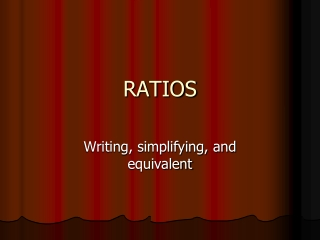DownloadDownload PresentationRATIOS

# RATIOS

Télécharger la présentation## RATIOS

- - - - - - - - - - - - - - - - - - - - - - - - - - - E N D - - - - - - - - - - - - - - - - - - - - - - - - - - -
##### Presentation Transcript

1. RATIOS Writing, simplifying, and equivalent

2. Ratios • Is a comparison of two quantities There are three ways to write a ratio: Example Statement- 6 cups party mix to 2 cups pretzels. 6 to 2 6 : 2 6 2

3. Equivalent Ratios • Two ratios that name the same number • Find equivalent ratios by multiplying or dividing each term by the same nonzero number. Example- 4:6= 4 ÷ 2 and 6 ÷ 2 = 2:3 4:6= 4 x 2 and 6 x 2 = 8:12

4. Simplifying Ratios • Divide the terms of the ratio by their GCF Example- 8:12 GCF = 4 8 ÷ 4 and 12 ÷ 4 = 2:3